# Kerala Syllabus 7th Standard Maths Solutions Chapter 1 Adding Angles

## Kerala State Syllabus 7th Standard Maths Solutions Chapter 1 Adding Angles

Joining angles Textbook Page No. 8

Can you draw this angle?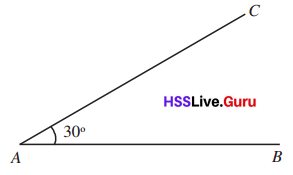Draw another angle on top like this:How many angles at A now?
∠CAB = _________
∠DAC = _________
∠DAC = 45°

Explanation: Given the two figures, one figure is 30° Angles.
The other figure is 45° and 30°.
Now, we will find the angles of ∠CAB and ∠DAC.
Observe the figure properly, then we find it easily.
The angle between C, A, and B is 30°.
Next, the angle between D, A, and C is 45°.
So, the ∠CAB = 30°, and ∠DAC = 45°

Do you see one more large angle?
How much is it?
∠DAB = _______
How did you compute it?
∠DAB = 45° + 30° = 75°
In the figures below, the measures of two angles are shown. Write the third angle as a sum or difference and compute its measure: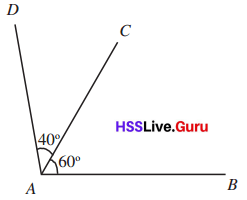∠DAB = ____ + _____ = ______

Explanation: Given the figure with two angles.
In between A, B, C, and D, we have two angles.
One angle is 60° and the other angle is 40°.
In a given question in between the angles, we have an addition symbol.
So, we find the third angle. We can add two angles we get the third angle.
so, the angles is 60° + 40° , ∠DAB = 100°.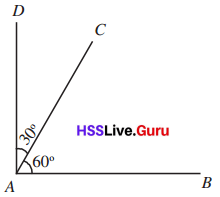∠DAB = ____ + _____ = ______

Explanation: Given the figure with two angles.
In between A, B, C, and D, we have two angles.
One angle is 30° and the other angle is 60°.
In a given question in between the given angles, we have an addition symbol.
So, we find the third angle. We can add two angles we get the third angle.
so, the angles is 30° + 60° , ∠DAB = 90°.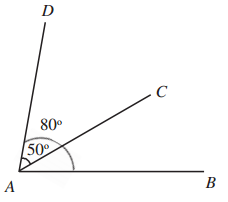∠CAB = ____ – _____ = ______

Explanation: Given the figure with two angles.
In between A, B, C, and D, we have two angles.
The angle between D, A, and B is 80° and the angle between D, A, and C is 5`0°.
In the given question in between the values, we have a subtraction symbol.
So, we find the third angle. We can subtract two angles we get the third angle.
Thus the angle is 80° –  50° = 30°, ∠CAB = 30°.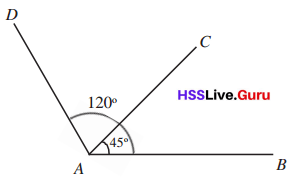∠DAC = ____ – _____ = ______

Explanation: Given the figure with two angles.
In between A, B, C, and D, we have two angles.
The angle between D, A, and B is 120° and the angle between C, A, and B is 45°.
In the given question, we have a subtraction symbol.
So, we find the third angle. We can subtract two angles we get the third angle.
Thus, the angle is 120° –  45° = 75°, ∠DAC = 75°.On both sides Textbook Page No. 9

Draw a line and a perpendicular to it as below: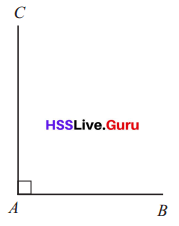Now draw an angle within it like this: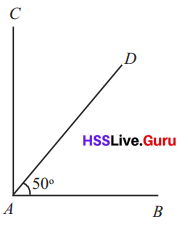What is the measure of ∠DAC?
∠DAC = ____ – _____ = _____

Explanation: Given the figure,
In between D, A, and B, we have a 50°angle.
Now, we find the angle of DAC.
The given figure is the right angle which has an angle of 90°, so the angle CAB = 90°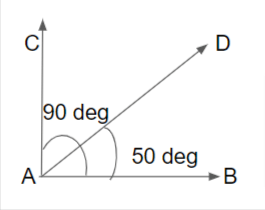In a given question, we have a subtraction symbol.
So, we find the third angle. We can subtract two angles we get the third angle.
Thus, the angle is 90° –  50° = 40°, ∠DAC = 40°.

Let’s stretch AB a bit to the left:How much is ∠DAE?
∠DAE = ____ + _____ = _____

Explanation: Given the figure, we have two angles.
In between D, A, and B, we have a 50°angle.
In between C, A, and D we have a 40°angle.
Now, we find the angle of DAE.
The given figure is a right angle which has an angle of 90°. So, the angle CAE = 90°
In a given question, we have an addition symbol.
So, we find the third angle. We can add two angles 90° and 40°. We get the third angle.
Thus, the angle is 90°+ 40° = 130°, ∠DAE = 130°.

Do you see how ∠DAE is related to ∠DAB?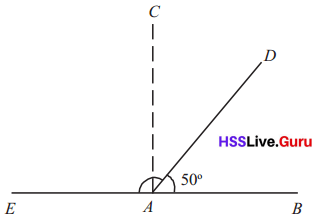Now look at this figure:Can you compute ∠DCA?

Explanation: Given the two figures, with an angle.
In between D, A, and B, we have a 50°angle.
In between D, C, and B, we have a 60°angle.
In a given question, it mentioned how the angle of DAE is related to DCA.
So, in the above question, the DAE value is 130°.
DAE + DAB = 130° + 50°= 180°
Now, we will find the value of DCA.
So, subtract the DCB value from 180°.
i.e., 180° – 60° = 120°.
The angle value of DCA = 120°

How about drawing a perpendicular at C and splitting this angle?How much is ∠DCE?
So, how much is ∠DCA?
∠DCE = 90° – 60° = 30°
∠DCA = 90° + 30° = 120°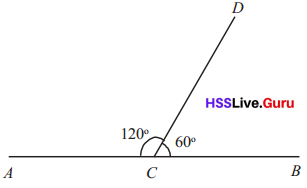Like this, can you compute the angle on the right in the figure below?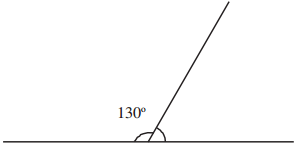Answer: Given the figure, with an angle.
In between D, C, and A, we have a 130°angle.
Linear pairs are angles formed in a line, their sum is equal to 180°.
Now, we will find the value of DCB.
So, subtract the DCA value from 180°.
i.e., 180° – 130° = 50°.
The angle value of DCB = 50°

In the figures below, the angles made on either side by joining two lines are shown; the measure of one angle is given. Compute the measure of the other:

(i)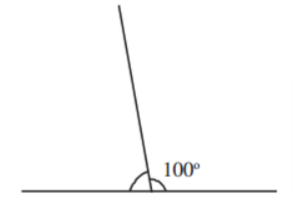What do we see in all these?

Answer: Given the figure, with an angle.
The given angle is 100°
Linear pairs are angle formed in a line, and their sum is equal to 180°.
Now, we will find the value of the angle.
So, subtract 100°value from 180°.
i.e., 180° – 100° = 80°.
Therefore, the angle value is 80°.

(ii)What do we see in all these?

Answer: Given the figure, with an angle.
The given angle is 40°
Linear pairs angle formed in a line, their sum is equal to 180°.
Now, we will find the value of the angle.
So, subtract 40°value from 180°.
i.e., 180° – 40° = 140°.
Therefore, the angle value is 140°.

(iii)What do we see in all these?

Answer: Given the figure, with an angle.
The given angle is 50°
Linear pairs angle formed in a line, their sum is equal to 180°.
Now, we will find the value of the angle.
So, subtract 50° value from 180°.
i.e., 180° – 50° = 130°.
Therefore, the angle value is 130°.

(iv)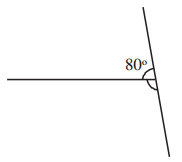What do we see in all these?

Answer: Given the figure, with an angle.
The given angle is 80°
Linear pairs angle formed in a line, their sum is equal to 180°.
Now, we will find the value of the angle.
So, subtract the 80° value from 180°.
i.e., 180° – 80° = 100°.
Then, the angle value is 100°.

If a line is drawn from another line, then the sum of the angles on either side is 180°.
A pair of angles that got like this is called a linear pair.Angle calculation Textbook Page No. 10

• How much is ∠ACE in this figure?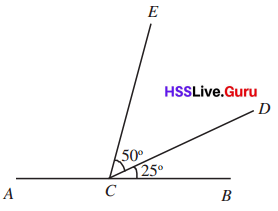Answer: The angle value of ∠ACE is 105°.

Explanation: Given that,
The value of ∠BCD = 25°.
The value of ∠DCE = 50°.
Now we will find the value of ∠ACE.
All these angles are in a line. So their sum is 180°.
So, ∠BCD + ∠DCE + ∠ACE = 180°.
25°+ 50° + ∠ACE = 180°.
75° + ∠ACE = 180°.
∠ACE = 180°-75°.
∠ACE = 105°.
So, the angle value of ∠ACE is 105°.

• How much is the angle between the two slanted lines in this figure?Answer: The angle between two slanted lines DBE is 75°.

Explanation: Given the figure. The below is the given with angles.Now, we will find the angle value of ∠DBE.
So, the angle value of ∠ABD = 45°
The angle value of ∠CBE = 60°.
We know that these angles are in a line. So the sum is 180°.
So, the value of ∠DBE is,
∠ABD + ∠CBE + ∠DBE = 180°.
45° + 60° + ∠DBE = 180°.
∠DBE = 180°- 45°- 60°
∠DBE = 75° .
So, the angle between two slanted lines DBE is 75°.

• In the figure below, ∠ACD = ∠BCE. How much is each?Answer: The angle of each is 105°.

Explanation: Given the figure,
The value of ∠ECD= 30°.
The value of ∠ACD and the value of ∠BCE is equal. Because both have the same angle distance.
So, ∠ACE = ∠BCD.
Here are the angles ∠ECD, ∠ACE, ∠and BCD in a line. So their sum is 180°.
∠ACE + ∠ECD + ∠BCD = 180°.
∠ACE = ∠BCD.
So, 2 ∠ACE + 30°   = 180°.
2 ∠ACE = 180°- 30°
2 ∠ACE = 150°.
∠ACE = 150°/2 = 75°
Now, the value of ∠ACE = 75°+ 30° = 105°.
So, ∠ACE = ∠BCD (∠BCD = 75°+ 30° = 105°).
Hence, the angle of each is 105°.

Cutting across Textbook Page No. 11

In the figure below, how much is the angle on the left?Suppose we extend the upper line downwards:Now there are two more angles underneath.
How much is each?
The angles at the top and bottom, on the left of the slanted line, form a linear pair.
There is such a linear pair on the right also.
Now, can’t we compute these angles?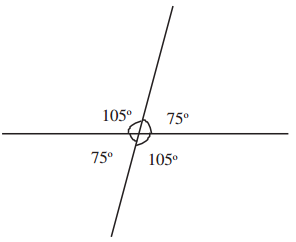In the figure below also, two lines cut across each other. Can you compute the other three angles marked?What do we see from all these?

Among the four angles made by two lines cutting across each other, the sum of each pair of nearby angles is 180°. Each pair of opposite angles are equal.

Now, can’t you calculate the angles marked in each of the figures below? Write them in the figure.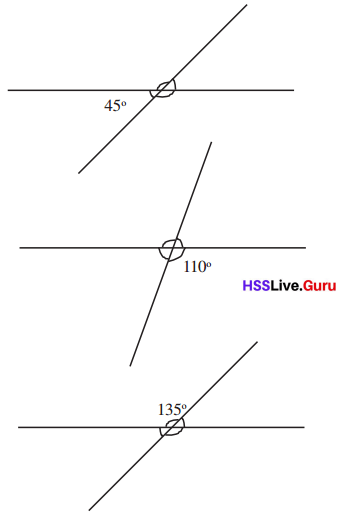Let’s do it! Textbook Page No. 12

In each figure, some angles are given. Find all other angles.

Question 1.

i.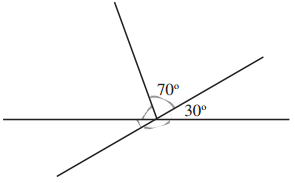Answer: The other angles are 150°, 80°, and 30°.

Explanation: Given the figure,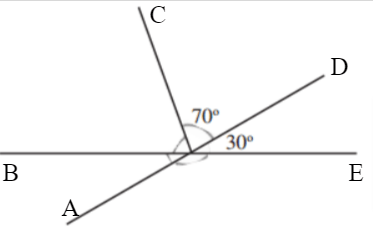The value of ∠EOD= 30°.
The opposite angles are equal. So, ∠EOD = ∠AOB = 30°.
Here are the angles ∠BOC, ∠COD, ∠and DOE in a line. So their sum is 180°.
∠BOC + ∠COD + ∠DOE  = 180°.
∠BOC + 70°+ 30°  = 180°.
∠BOC = 180°-70°- 30°
∠BOC = 80°.
Next, the angles ∠AOE = ∠BOD. Because both are opposite angles.
So,  ∠BOD = ∠BOC+∠COD
∠BOD = 80°+70° = 150°.
The other angles are 150°, 80°, and 30°.

ii.Answer: The other angles are 50°, 130°, and 60°.

Explanation: Given the figure,The value of ∠COD= 50°.
The opposite angles are equal. So, ∠COD = ∠AOB = 50°.
Here are the angles ∠of BOC, ∠and COD in a line. So their sum is 180°.
∠BOC + ∠COD = 180°.
∠BOC + 50°  = 180°.
∠BOC = 180°-50°
∠BOC = 130°.
Next, the angles ∠AOE, ∠EOD, ∠and DOC are in a line. So their sum is 180°.
∠AOE + ∠EOD + ∠DOC  = 180°.
∠EOD + 70°+ 50°  = 180°.
∠EOD = 180°-70°-50°
∠EOD = 60°.
The other angles are 50°, 130°, and 60°.

iii.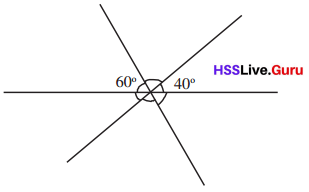Answer: The other angles are 60°, 80°, and 80°.

Explanation: Given the figure,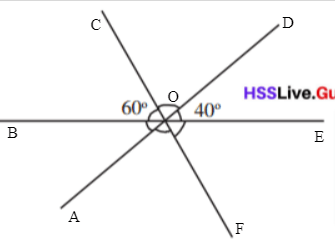The value of ∠COB= 60°.
The opposite angles are equal. So, ∠COB = ∠EOF = 60°.
The value of ∠DOE= 40°.
Here are the angles ∠BOC, ∠COD, ∠and DOE in a line. So their sum is 180°.
∠BOC + ∠COD + ∠DOE= 180°.
∠COD + 60° + 40° = 180°.
∠COD = 180°-60°- 40°
∠COD = 80°.
The angles ∠COD = ∠AOF = 80°. Because both are opposite angles.
The other angles are 60°, 80°, and 80°.

iv.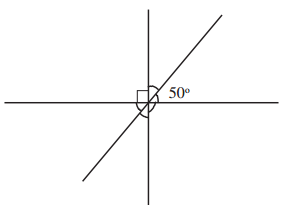Answer: The other angles are 90°, 50°, 40°, and 40°.

Explanation: Given the figure,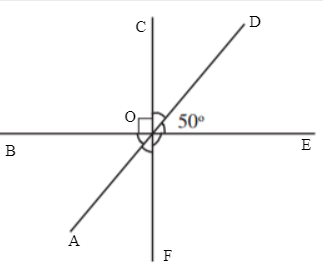The value of ∠COB= 90°.
The opposite angles are equal. So, ∠COB = ∠EOF = 90°.
The value of ∠DOE= 50°.
∠DOE = ∠BOA= 50°. Because these are opposite angles.
Here are the angles ∠BOC, ∠COD, ∠and DOE in a line. So their sum is 180°.
∠BOC + ∠COD + ∠DOE= 180°.
∠COD + 90° + 50° = 180°.
∠COD = 180°-90°- 50°
∠COD = 40°.
Next, the angles ∠COD = ∠AOF  = 40°. Because both are opposite angles.
Hence, the other angles are 90°, 50°, 40°, and 40°.

error: Content is protected !!